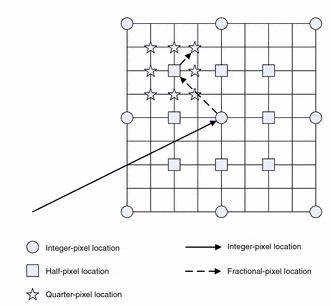# Interpolation-Free Fractional-Pixel Motion Estimation Algorithms with Efficient Hardware Implementation`

## Abstract

This paper presents interpolation-free fractional-pixel motion estimation (FME) algorithms and efficient hardware prototype of one of the proposed FME algorithms. The proposed algorithms use a mathematical model to approximate the matching error at fractional-pixel locations instead of using the block matching algorithm to evaluate the actual matching error. Hence, no interpolation is required at fractional-pixel locations. The matching error values at integer-pixel locations are used to evaluate the mathematical model coefficients. The performance of the proposed algorithms has been compared with several FME algorithms including the full quarter-pixel search (FQPS) algorithm, which is used as part of the H.264 reference software. The computational cost and the performance analysis show that the proposed algorithms have about 90% less computational complexity than the FQPS algorithm with comparable reconstruction video quality (i.e., approximately 0.2 dB lower reconstruction PSNR values). In addition, a hardware prototype of one of the proposed algorithms is presented. The proposed architecture has been prototyped using the TSMC 0.18 μm CMOS technology. It has maximum clock frequency of 312.5 MHz, at which, the proposed architecture can process more than 70 HDTV 1080p fps. The architecture has only 13,650 gates. The proposed architecture shows superior performance when compared with several FME architectures.

M. Sayed, W. Badawy, and G. Jullien, “Interpolation-Free Fractional-Pixel Motion Estimation Algorithms with Efficient Hardware Implementation,” the Journal of Signal Processing Systems. Volume 67, Issue 2 , pp 139-155, May 2012.
Link to the list of other Peer Journal Publications